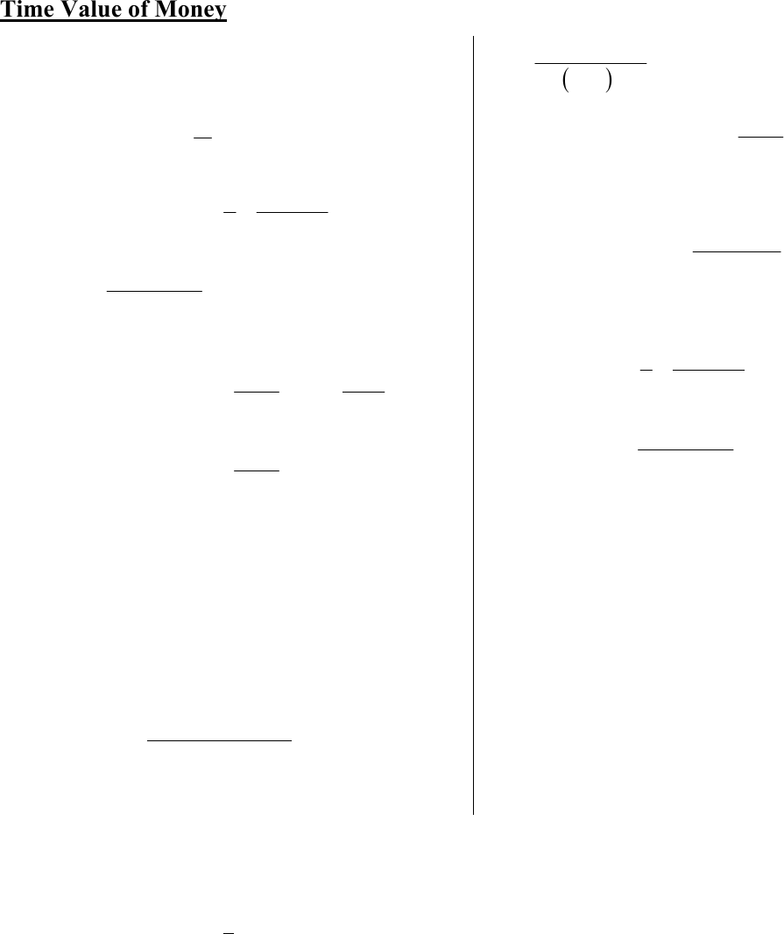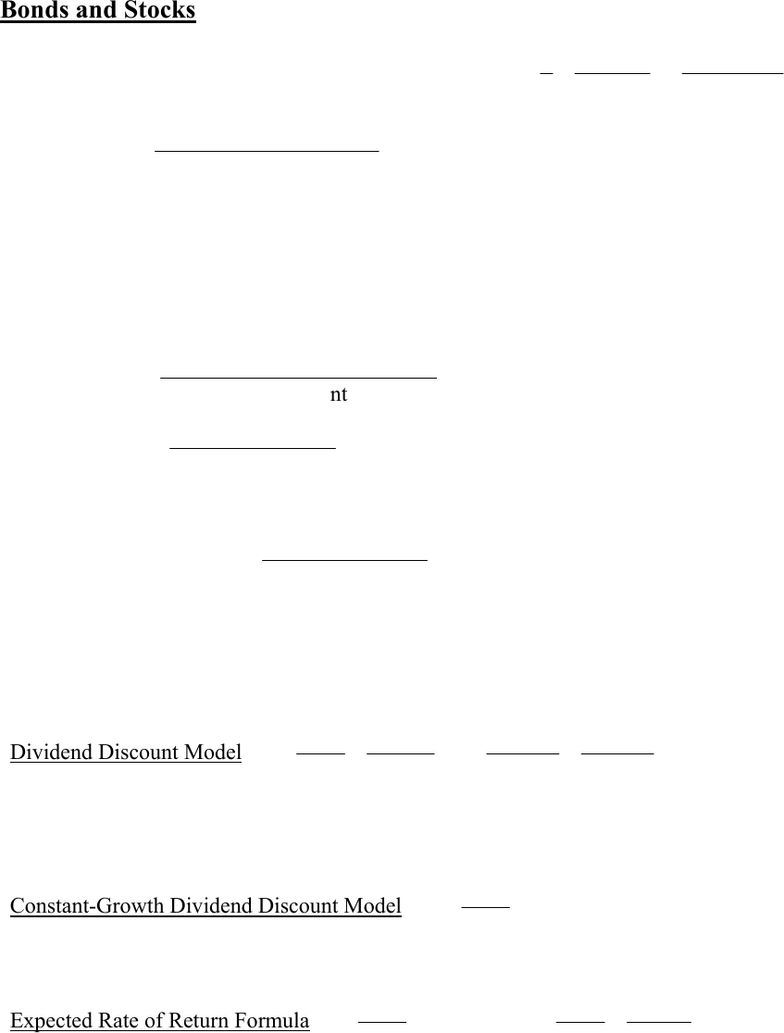Study Guides (390,000)
CA (150,000)
York (10,000)
Final

# ADMS 3530 Final: 3530 Final Exam Formula Sheet.F18Exam

Department
Course Code
Professor
Alagurajah
Study Guide
Final

This preview shows pages 1-2. to view the full 6 pages of the document.1
Final Exam Formula Sheet
Time Value of Money
FV =

t
r1Investment PV =

t
r1
Value Future
PV of a perpetuity =
r
C PV of a growing perpetuity = gr
C
1
PV of an annuity =
t
rr
r
C)1(
11
=
r
r
C
t
)1(1 (easier to calculate)
FV of an annuity =
r
r
C
t1)1(
PV (Growing Annuity) =
t
r
g
gr
C
1
1
1
1
FV (Growing Annuity) =
 

tt gr
gr
C
11
1
Annuity factor =
t
rr
r)1(
11
=
r
rt
)1(1
(lower version is easier to calculate)
PV (Annuity Due) = (1+r) x (PV of an annuity)
FV (Annuity Due) = (1+r) x (FV of an annuity)
1 + Real rate = rateInflation 1
rate Nomimal1
APR = Period Rate m
EAR =

1Rate Period1 m
Period Rate = 1)EAR1(
1
m
where m = number of periods per year

Only pages 1-2 are available for preview. Some parts have been intentionally blurred.2
Bonds and Stocks
Price of a bond = PV (Coupons) + PV (Face Value) = tt rrr
r
C)1(
Value Face
)1(
11
Current yield = price Bond
paymentCoupon Annual
Yield to maturity (YTM) = interest rate for which the present value of the bond’s
payments equals the price
Rate of return = Investmen
t
change Price incomeCoupon
Dividend yield = priceStock
payment Dividend
Price earnings (P/E) ratio = shareper Earnings
PriceStock
Sustainable growth rate g = ROE Plowback ratio
Dividend Discount Model: H
H
Hr
P
rr
r
P)1()1(
DIV
...
)1(
DIV
1
DIV H
2
21
0
where H is the horizon date, and PH is the expected price of the stock at date H
Constant-Growth Dividend Discount Model: gr
P
1
0
DIV
Expected Rate of Return Formula: g
P
r
0
1
DIV or
0
01
0
1
DIV
P
PP
P
r